Select Page

Economic Order Quantity (EOQ)

Economic Order Quantity is the level of inventory that minimizes the total inventory holding costs and ordering costs. It is one of the oldest classical production scheduling models. Economic order quantity refers to that number (quantity) ordered in a single purchase so that the accumulated costs of ordering and carrying costs are at the minimum level. In other words, the quantity that is ordered at one time should be so, which will minimize the total of. Cost of placing orders and receiving the goods, and Cost of storing the goods as well as interest on the capital invested.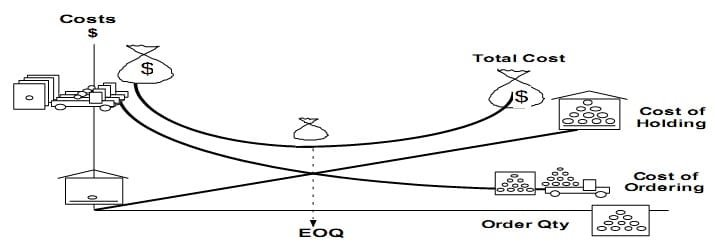The economic order quantity can be determined by the following simple formula:

Formula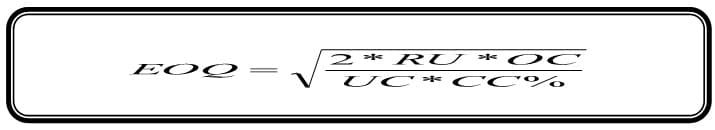EOQ = Economic Order Quantity,

RU = Annual Required Units,

OC    = Ordering Cost for one Unit

UC = Inventory Unit Cost,

CC = Carrying Cost as %age of Unit Cost

>> Practice Economic Order Quantity Problems and Solutions

Example 1:

Demand for the Child Cycle at Best Buy is 500 units per month. Best Buy incurs a fixed order placement, transportation, and receiving cost of Rs. 4,000 each time an order is placed. Each cycle costs Rs. 500 and the retailer has a holding cost of 20 percent. Evaluate the number of computers that the store manager should order in each replenishment lot?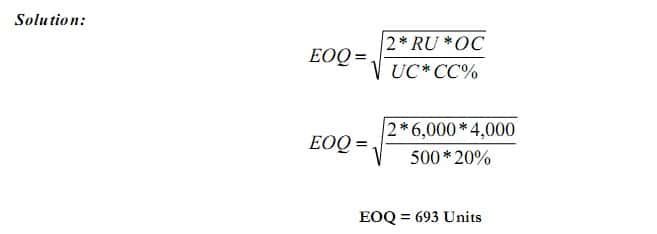>> Practice Economic Order Quantity Problems and Solutions

Example 2:

ABC Ltd. uses EOQ logic to determine the order quantity for its various components and is planning its orders. The Annual consumption is 80,000 units, Cost to place one order is Rs. 1,200, Cost per unit is Rs. 50 and carrying cost is 6% of Unit cost. Find EOQ, No. of order per year, Ordering Cost and Carrying Cost and Total Cost of Inventory.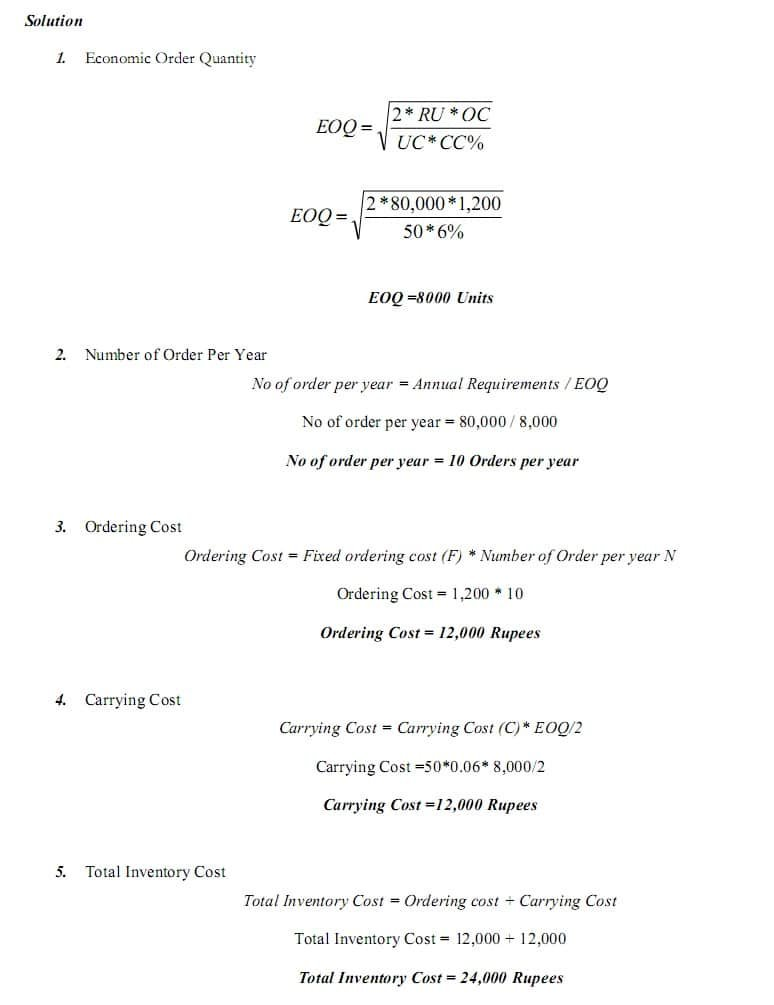>> Question and Answers:  Economic Order Quantity Problems and Solutions

Example 3:

Midwest Precision Control Corporation is trying to decide between two alternate Order Plans for its inventory of a certain item. Irrespective of the plan to be followed, demand for the item is expected to be 1,000 units annually. Under Plan 1st, Midwest would use a teletype for ordering; order costs would be Rs. 40 per order. Inventory holding costs (carrying cost) would be Rs. 100 per unit per annum. Under Plan 2nd order costs would be Rs. 30 per order. And holding costs would 20% and unit Cost is Rs. 480. Find out EOQ and Total Inventory Cost than decide which Plan would result in the lowest total inventory cost?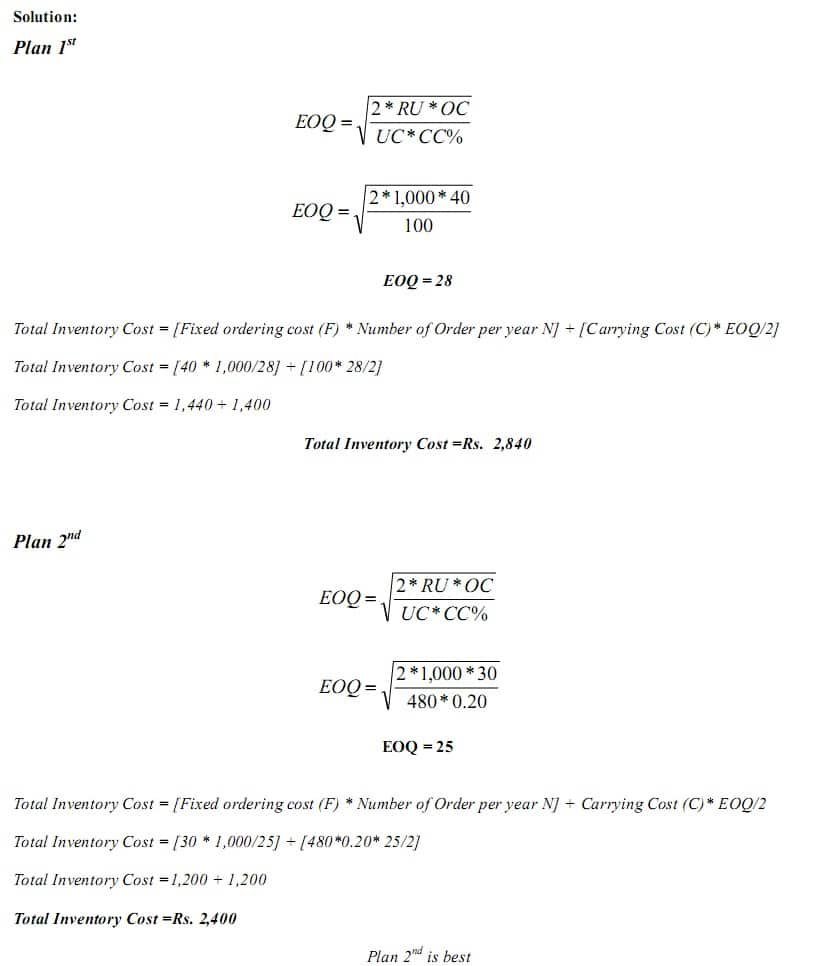>> Practice Economic Order Quantity Problems and Solutions

Example 4:

A local TV repairs shop uses 36,000 units of a part each year (A maximum consumption of 100 units per working day). It costs Rs. 20 to place and receive an order. The shop orders in lots of 400 units. It cost Rs. 4 to carry one unit per year of inventory.

Requirements:

(1) Calculate total annual ordering cost

(2) Calculate total annual carrying cost

(3) Calculate total annual inventory cost

(4) Calculate the Economic Order Quantity

(5) Calculate the total annual cost inventory cost using EOQ inventory Policy

(6) How much save using EOQ

(7) Compute ordering point assuming the lead time is 3 days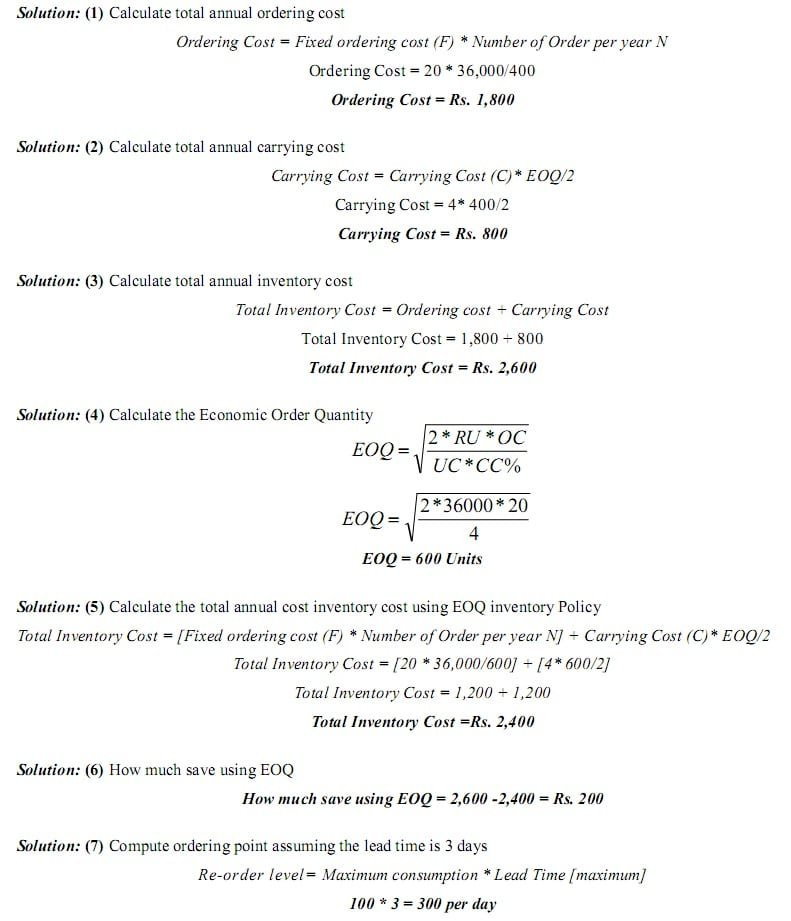>> Practice Economic Order Quantity Problems and Solutions